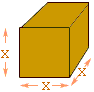Who is asking: Student Level: Middle Question: if the volume of a cube is 64 cm, then what's the total surface area? I also want to know how to show my work. Hi, First I would draw a diagram of a cube and label it.It is a cube so the length, width and height are all equal. I called this number x cm. The surface of the cube is composed of 6 identical faces, each x cm by x cm. The volume is x3 cubic centimeters, but you say the volume is 64 cubic centimeters. So what is x? What is the area of each face? What is the total surface area? Penny Go to Math Central